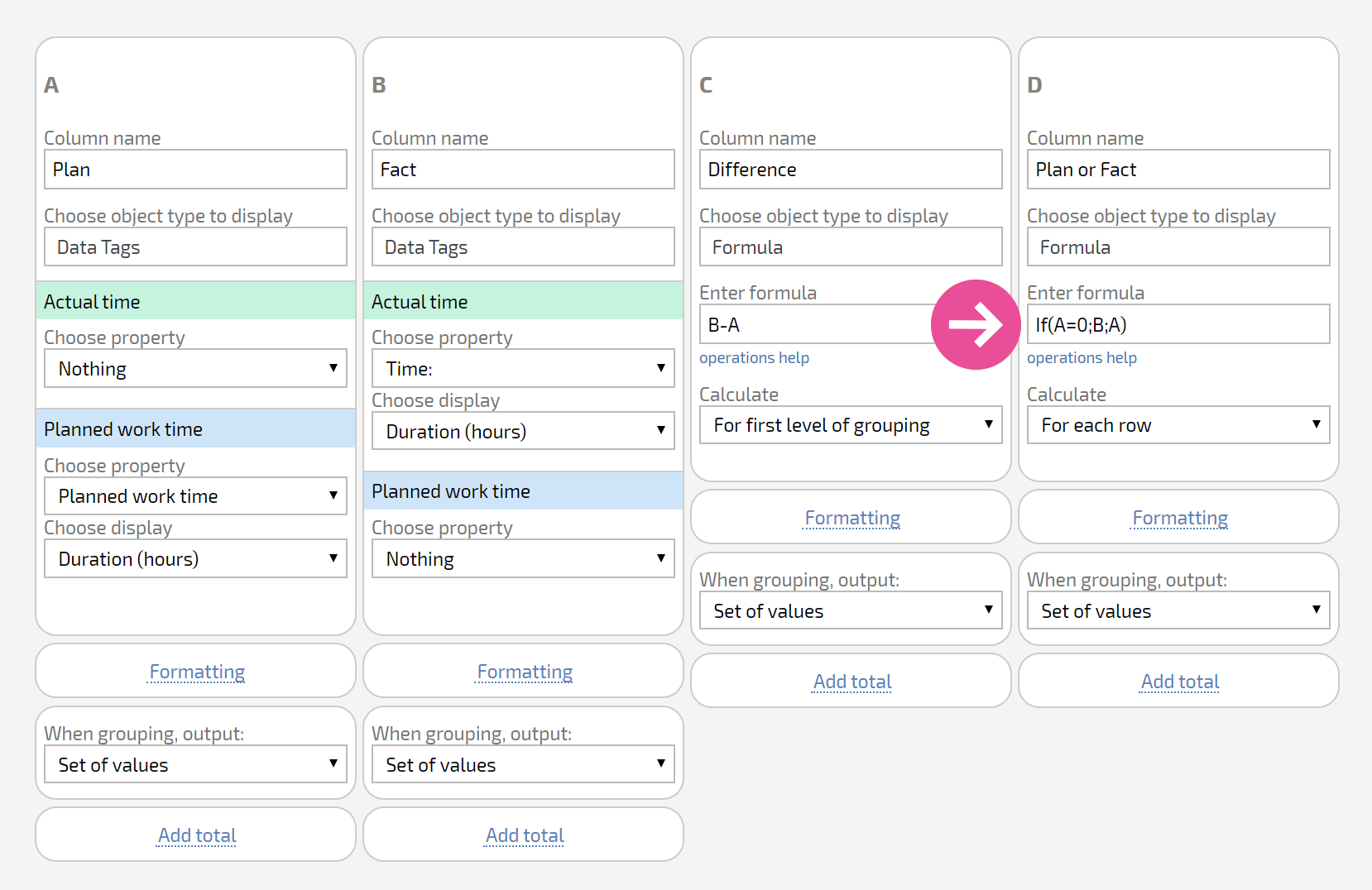# IF Function

This function returns one of two values, depending on a condition.

Format:

• IF(condition;yes_value;no_value)

where:

• condition - logical expression using the symbols >, <, =, >=, <=, !=, another function or value
• yes_value - number or string that the function returns if the condition is met
• no_value - number or string that the function returns if the condition is not met

Examples:

IF(D > 100500;"A lot";"A little")

IF(CONTAIN(L;"Qualification");G;"")

Notes:

• the condition can be an arithmetic expression. If the result of this expression is a non-zero number, then the condition is considered to be fulfilled. If the result of the expression is zero, then the condition is considered not fulfilled
• the values can be expressions that use data from report columns or variables in calculated fields
• use a "Yes"/"No" test to check a Checkbox custom field in a data tag or data tag calculated field. In other cases, without data tags, use a 1/0 check.

## Particularities of working with formulas and functions

• All arguments of the IF formula must be included (Condition;Action_IF_YES;Action_IF_NO);
• Nesting IF inside IFs is not limited, but the number of arguments must be correct;
• Empty NUMBER fields equal zero, and nothing more;
• Empty STRING fields and other fields (or not all) are entered as empty quotes: "";
• You can multiply by "-1";
• Line breaks cannot be used in formulas or when generating documents.

Example:

Depending on whether or not there is a number in column D, either return either that number or the number in column E: